# Simplevis

#### 2022-03-04

``````library(dplyr)
library(simplevis)
library(palmerpenguins)
library(ggplot2)
library(patchwork)
set.seed(123456789)``````

### Purpose

`simplevis` is a package of `ggplot2` and `leaflet` wrapper functions that aims to make visualisation easier with less brainpower required.

### Visualisation families

`simplevis` supports many visualisation family types.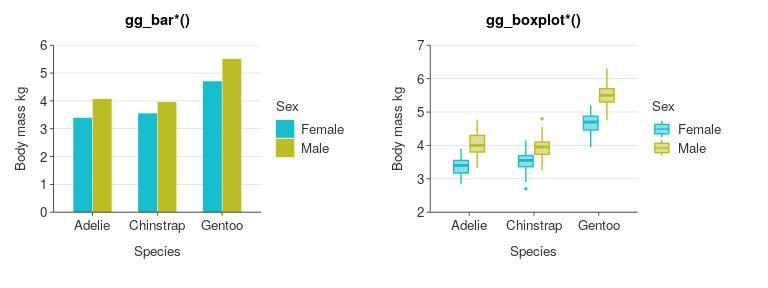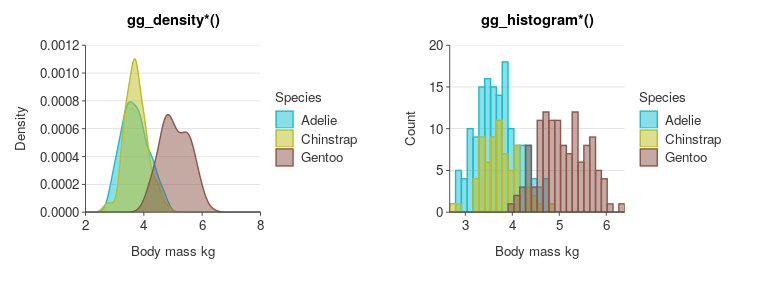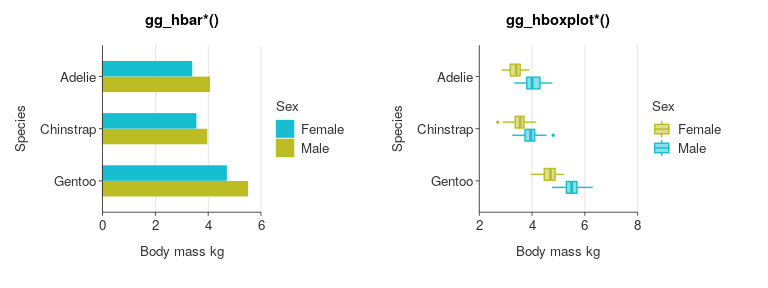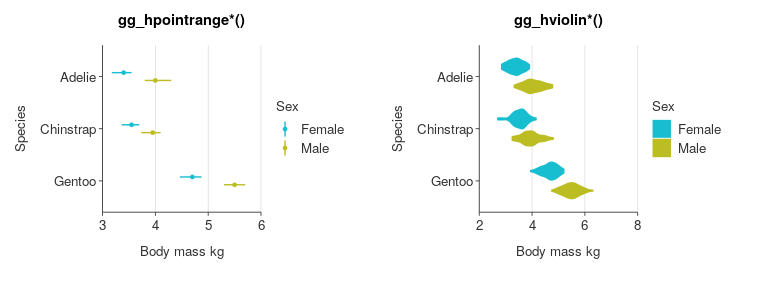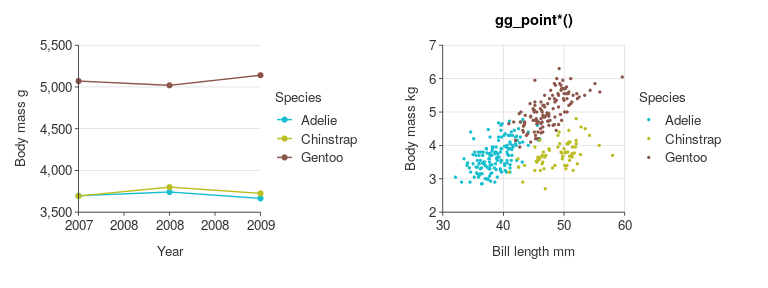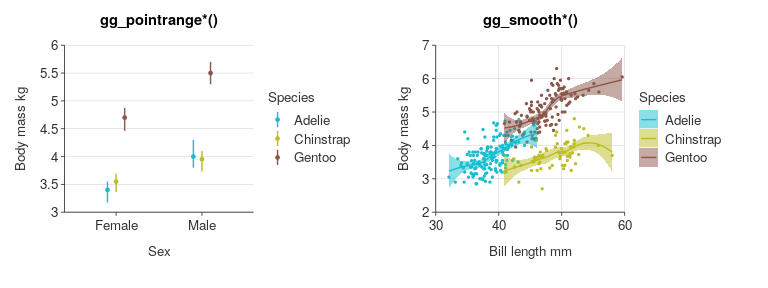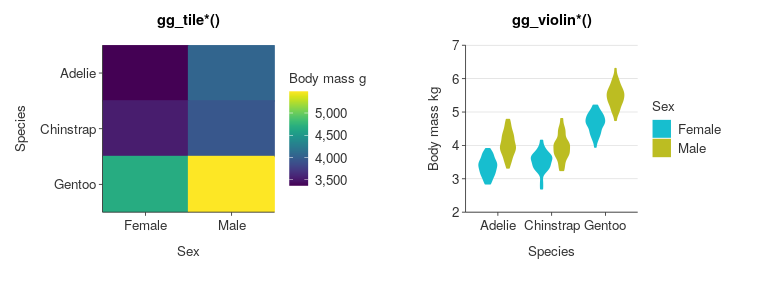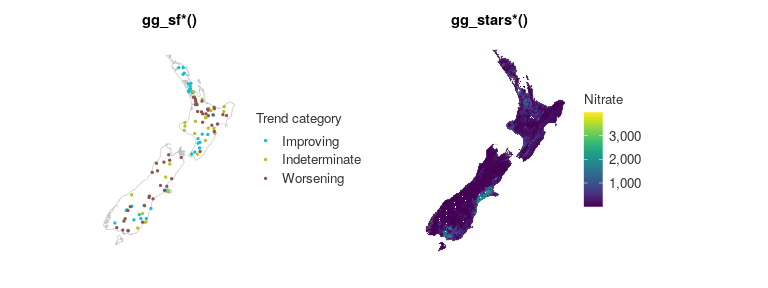### 4 functions per family: colouring, facetting, neither or both

Each visualisation family generally has four functions.

This is based on whether or not a visualisation is to be:

• not coloured by a variable and not facetted (`*()`)
• coloured by a variable, but not facetted (`*_col()`)
• facetted, but not coloured by a variable (`*_facet()`)
• coloured by a variable and facetted (`*_col_facet()`)

The premise is that these are the most common types of visualisation for each of the families.

All arguments for variables are required unqutoted and follow an `*_var` format (i.e. `x_var`, `y_var`, `col_var` and `facet_var`)

For example, code below shows these combinations with code and output for the `gg_point*()` family.

``````gg_point(penguins,
x_var = bill_length_mm,
y_var = body_mass_g)``````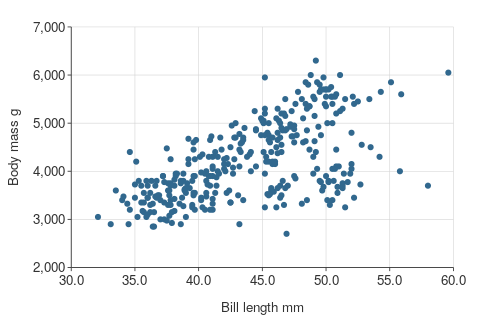``````gg_point_col(penguins,
x_var = bill_length_mm,
y_var = body_mass_g,
col_var = sex)``````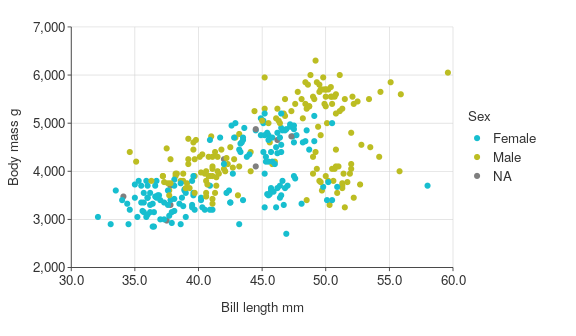``````gg_point_facet(penguins,
x_var = bill_length_mm,
y_var = body_mass_g,
facet_var = species)``````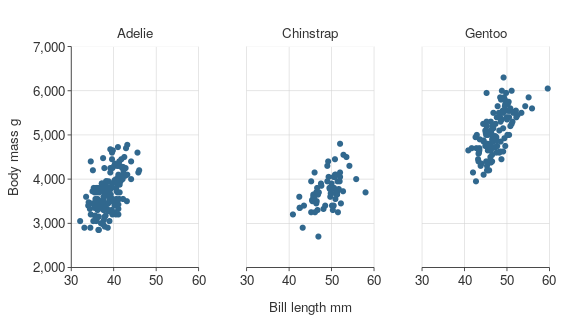``````gg_point_col_facet(penguins,
x_var = bill_length_mm,
y_var = body_mass_g,
col_var = sex,
facet_var = species)``````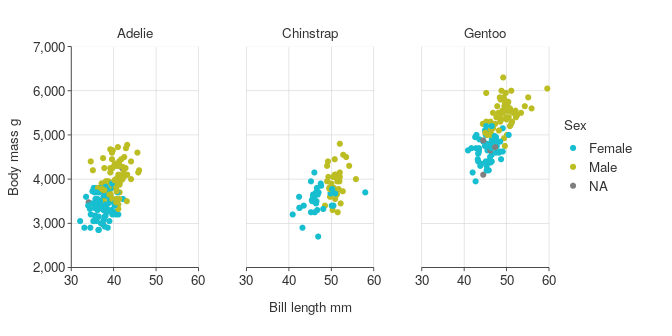### Customising plots

simplevis plots are designed to work and look clean with the bare minimum of code.

However, there is plenty of flexibility to customise.

#### Colour

Change the colour palette by supplying a vector of hex code colours to the `pal` argument.

``````gg_point_col(penguins,
x_var = bill_length_mm,
y_var = body_mass_g,
col_var = species,
pal = c("#da3490", "#9089fa", "#47e26f"))``````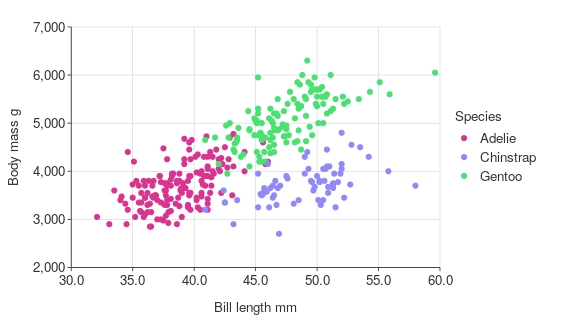Numerous colour palettes are available from the `pals` package.

simplevis also supports easy colouring of numeric variables for `gg_point*()`, `gg_bar*()`, `gg_hbar*()`, `gg_sf*()` and `gg_stars*()` (and equivalent leaflet functions).

The col_method argument allows you to specify the method for colouring with a default of continuous.

``````gg_point_col(penguins,
x_var = bill_length_mm,
y_var = body_mass_g,
col_var = flipper_length_mm,
col_method = "continuous",
subtitle = "col_method = 'continuous'")``````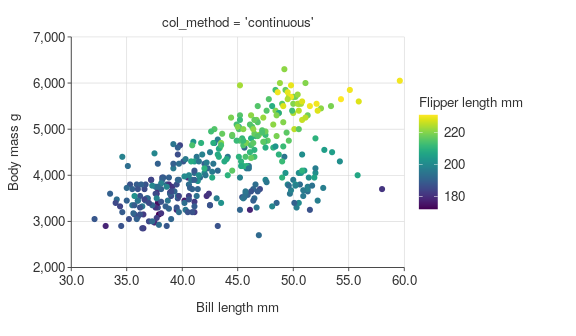Other methods available are `bin` and `quantile`.

For `bin` and `quantile`, you can fine-tune using the `col_breaks_n` or `col_cuts` arguments.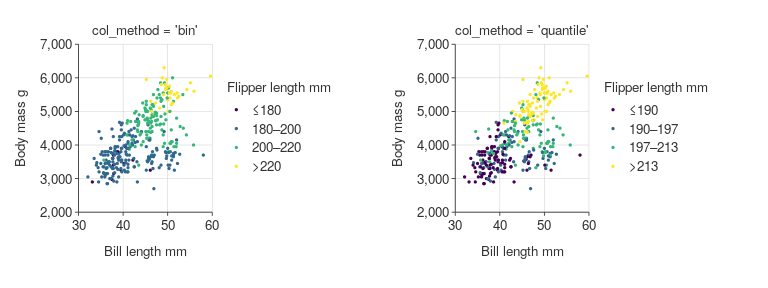You can also adjust the opacity of objects in the visualisation through the `alpha_*()` arguments.

Refer to the colour article for further information.

#### Themes

You can adjust the theme of any `simplevis` plot by providing a `ggplot2` theme to the `theme` argument.

``````gg_point_col(penguins,
x_var = bill_length_mm,
y_var = body_mass_g,
col_var = species,
title = "A nice title",
subtitle = "And a subtitle",
theme = ggplot2::theme_grey())``````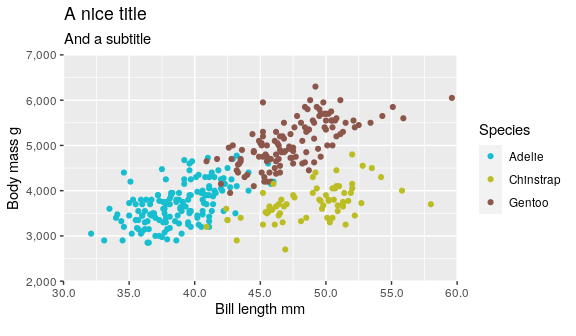You can also create your own quick themes with the `gg_theme()` function.

``````custom_theme <- gg_theme(
pal_body = "white",
pal_title = "white",
pal_subtitle = "white",
pal_background = c("#232323", "black"),
pal_gridlines = "black",
gridlines_h = TRUE,
gridlines_v = TRUE)

gg_point_col(penguins,
species,
x_var = bill_length_mm,
y_var = body_mass_g,
theme = custom_theme)``````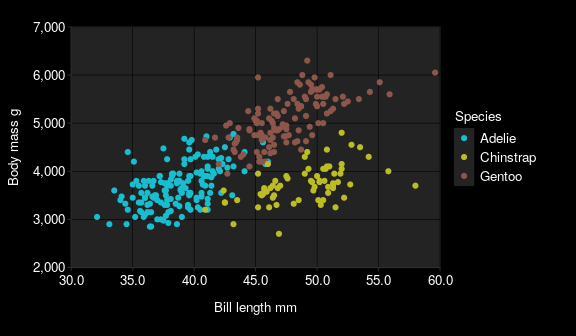Refer to the themes article for further information.

#### Consistent prefixes

There are lots of arguments available to modify the defaults.

In general, arguments have consistent prefixes based on `x_*`, `y_*`, `col_*` or `facet_*`, and as such the autocomplete can help identify what you need.

Some examples of transformations available are:

• `*_na_rm` to quickly not include NA observations
• `*_labels` to adjust labels for any x, y, col or facet scale
• `*_zero` to start at zero for numeric x or y scales
• `*_breaks_n` for the number of numeric bins of breaks for the x, y or col scale to aim for
• `*_rev` to reverse the order of categorical x, y or col scales in bars
• `*_expand` to add padding to an x or y scale.
• `*_balance` to balance a numeric scale, so that zero is in the centre
• `col_legend_none` to turn the legend off.
``````plot_data <- storms %>%
group_by(year, status) %>%
summarise(wind = mean(wind))

gg_line_col(plot_data,
x_var = year,
y_var = wind,
col_var = status,
x_breaks_n = 10,
x_labels = function(x) stringr::str_sub(x, 3, 4),
y_labels = scales::label_dollar(accuracy = 1),
col_labels = c("H", "TD", "TS"),
y_zero = TRUE,
y_breaks_n = 10,
y_expand = ggplot2::expansion(add = c(0, 10)))``````